### Controlling Parallelism in Spark by controlling the input partitions by controlling the input partitions

It is useful to be able to control the degree of parallelism when using Spark. Spark provides a very convenient method to increase the degree of parallelism which should be adequate in practice. This blog entry of the "Inside Spark" series describes the knobs Spark uses to control the degree of parallelism.

## Controlling the number of partitions in the "`local`" mode

Spark is designed ground up to enable unit testing. To control the degree of parallelism in the local mode simply utilize the function
`JavaRDD<String> org.apache.spark.api.java.JavaSparkContext.parallelize(List<String> list, int numSlices)`
The following snippet of code demonstrates this usage-
```public class SparkControlPartitionSizeLocally {
public static void main(String[] args) {
JavaSparkContext sc =
new JavaSparkContext("local","localpartitionsizecontrol");
String input = "four score and seven years ago our fathers "
+ "brought forth on this continent "
+ "a new nation conceived in liberty and "
+ "dedicated to the propostion that all men are created equal";
List<String> lst = Arrays.asList(StringUtils.split(input, ' '));

for (int i = 1; i <= 30; i++) {
JavaRDD<String> rdd = sc.parallelize(lst, i);
System.out.println(rdd.partitions().size());
}

}
}
```
Towards the end of the iterations in the `for` loop, the number of partitions will be greater than the number of words. Consequently the resulting spark RDD will have some empty partitions.

## Controlling the number of partitions in the "`cluster`" mode

Next I will demonstrate how the degree of parallelism is defined in the server mode. But before I do that let us look at the classes Spark utilizes to perform its Input/Output functions.

### Classes participating in the Input/Output?"

Spark uses the same classes as Hadoop to perform its Input/Output. InputFormat/RecordReader class pair performs the Input duties and how the OutputFormat/RecordWriter class pair performs the Output duties in Hadoop.
In Chapter 7 of Pro Apache Hadoop 2nd Edition I have discussed the Input/Output framework of Hadoop in detail. I will skip that discussion here.

### Inside the JavaSparkContext.textFile(...)

Below are the relevant lines of code from the SparkContext.scala program which configures the Spark Input process.
```def textFile(path: String, minPartitions: Int = defaultMinPartitions): RDD[String] = {
assertNotStopped()
classOf[TextInputFormat],
classOf[LongWritable],
classOf[Text],minPartitions)
.map(pair => pair._2.toString).setName(path)
}
```
The call to `textFile` delegates to the `hadoopFile` function which utilizes the `TextInputFormat`. Note that it uses the old Hadoop API as on the old Hadoop API has the option to define the `minPartitions` which allows you to increase the partitions than the default. We will soon see that you cannot use this parameter to decrease the partitions to a value lower than the default number of partitions.
```def hadoopFile[K, V](path: String,
inputFormatClass: Class[_ <: InputFormat[K, V]],
keyClass: Class[K],
valueClass: Class[V],
minPartitions: Int = defaultMinPartitions): RDD[(K, V)] = {
assertNotStopped()
// A Hadoop configuration can be about 10 KB, which is pretty big,
val setInputPathsFunc = (jobConf: JobConf) =>
FileInputFormat.setInputPaths(jobConf, path)

inputFormatClass,
keyClass,valueClass,minPartitions).setName(path)
}
```

## Preparing the Dataset for Code Samples

Similar to my previous blog article on sampling large datasets, I will use the Airline dataset for writing code samples in this article.
Explode both of the above files in folder on your local machine. I exploded mine in the folder `C:/MyData/airlinedata/textdata`. You should have two files in this folder
1. `1987.csv` - Size = 124183 KB
2. `1988.csv` - Size = 489297 KB
We will explore Spark's Input/Output features using the above mentioned files.

## Demonstration

To demonstrate how number of partitions is defined at Spark runtime we will run the program depicted in the figure below. We will simulate the "server" mode in the "local" mode to demonstrate how the partition size (and consequently the number of partitions) is determined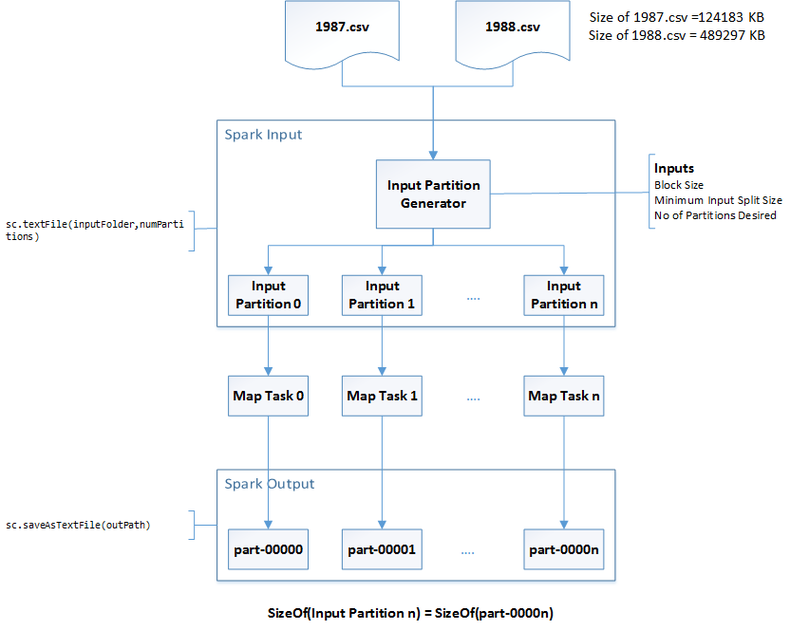### Key Parameters participating in the Partition definition process

The key parameters used to control the number of partitions are as follows
1. `dfs.block.size` - The default value in Hadoop 2.0 is 128MB. In the local mode the corresponding parameter is `fs.local.block.size` (Default value 32MB). It defines the default partition size.
2. `numPartitions` - The default value is 0 which effectively defaults to 1. This is a parameter you pass to the `sc.textFile(inputPath,numPartitions)` method. It is used to decrease the partition size (increase the number of partitions) from the default value defined by `dfs.block.size`
3. `mapreduce.input.fileinputformat.split.minsize` - The default value is 1 byte. It is used to increase the partition size (decrease the number of partitions) from the default value defined by `dfs.block.size`

### Partition Size Definition

The actual partition size is defined by the following formula in the method `FileInputFormat.computeSplitSize`
```package org.apache.hadoop.mapred;
/*Import Statements*/
public abstract class FileInputFormat<K, V> implements InputFormat<K, V> {
....
protected long computeSplitSize(long goalSize,
long minSize,
long blockSize) {
return Math.max(minSize, Math.min(goalSize, blockSize));
}
...
}
```
where,
`minSize` is the hadoop parameter `mapreduce.input.fileinputformat.split.minsize`
`blockSize` is the value of the `dfs.block.size` in cluster mode and `fs.local.block.size` in the local mode
`goalSize=totalInputSize/numPartitions`
where,
`totalInputSize` is the total size in bytes of all the files in the input path.
`numPartitions` is the custom parameter provided to the method `sc.textFile(inputPath, numPartitions)`

## Scenarios

Let us look at how the above mentioned parameters affect the number of partitions (or partition size). For this demonstration the input files and their sizes will be as follows
1. `1987.csv` - Size = 124183 KB
2. `1988.csv` - Size = 489297 KB
Since the demonstration is done in local mode we will be using the parameter `fs.local.block.size` in place of `dfs.block.size`

### Scenario 1 - Default

The default settings are
1. `fs.local.block.size` = 32 MB
2. `numPartitions` = 0 (this defaults to 1)
3. `mapreduce.input.fileinputformat.split.minsize` = 1 byte
The figure below shows the partitions and their sizes.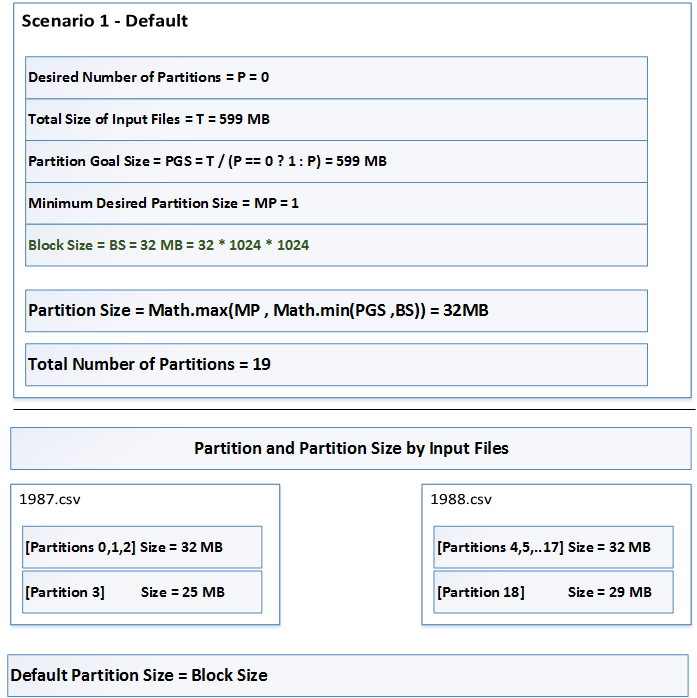The interesting this to notice here is that each partition belongs to one and only one file. Partition 3 is lower than 32MB because the last 25MB of the 1987.csv are contained in it. Likewise Partition 18 which is 29MB and contains the last block of 1988.csv

### Scenario 2 - Decrease Partition Size

The default settings are
1. `fs.local.block.size` = 32 MB
2. `numPartitions` = 30
3. `mapreduce.input.fileinputformat.split.minsize` = 1 byte
The figure below shows the partitions and their sizes.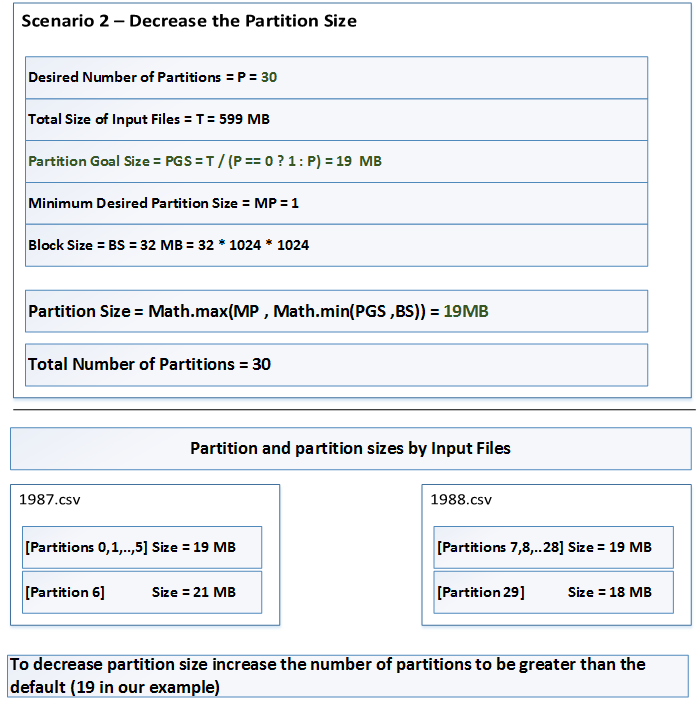The size of Partition 6 is interesting. It is 21MB. The first 1MB comes from last block of the 1987.csv and the remaining 20MB comes from the first block of the file 1988.csv. In Scenario 1, the partitions can contain entries from multiple files. This is done to respect the value of `numPartitions`

### Scenario 3 - Increasing Partition Size (the wrong way)

The default settings are
1. `fs.local.block.size` = 32 MB
2. `numPartitions` = 5 (lower than 19 as produced in Scenario 1)
3. `mapreduce.input.fileinputformat.split.minsize` = 1 byte
The figure below shows the partitions and their sizes.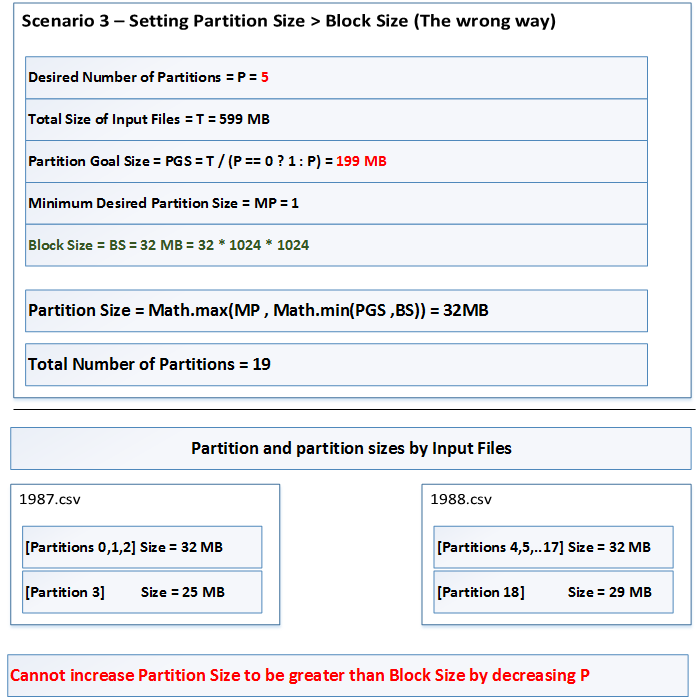Clearly we cannot increase partition size by decreasing the value of `numPartitions`. Scenario 4 demonstrates the correct way to increase partition size. There are no situations I know of where increasing partition size is a good idea, since it will reduce the degree of parallelism. Scenario 3 and Scenario 4 are only there is illustrate the knobs Spark and Hadoop utilizes to control partition sizes

### Scenario 4 - Increasing Partition Size (the correct way)

The default settings are
1. `fs.local.block.size` = 32 MB
2. `numPartitions` = 0
3. `mapreduce.input.fileinputformat.split.minsize` = 64 MB
The figure below shows the partitions and their sizes.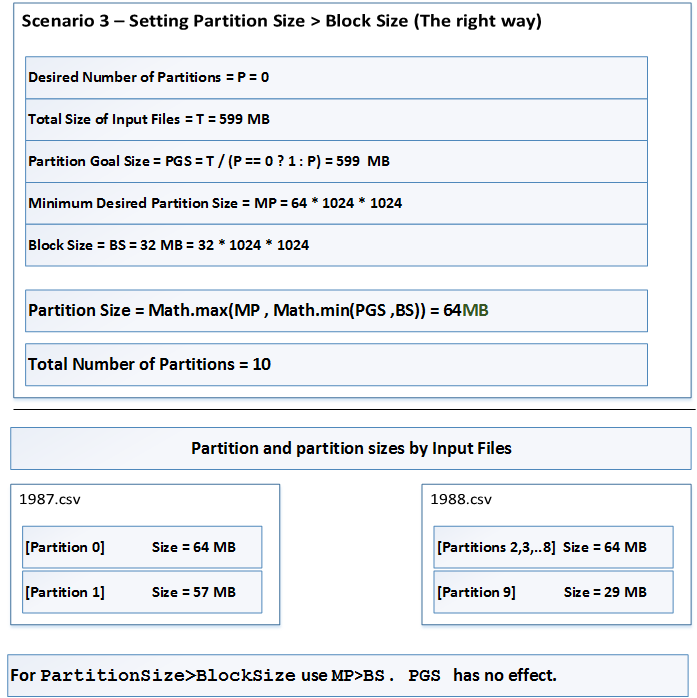## Demonstration Classes

The four scenarios above can be simulated by executing the following class from my
These are part of my Github project.
`com.axiomine.spark.examples.io.PrintSparkMapSidePartitionSizeControl`
It takes one parameter, `inputPath`. I have placed the two files (`1987.csv` and `1988.csv` in a folder `c:/mydata/airline/txt`). When invoked with the parameter `c:/mydata/airline/txt` the above 4 scenarios can be simulated. This class will only print the partition and their sizes.
The four scenarios can be executed by invoking the class
`com.axiomine.spark.examples.io.SparkMapSidePartitionSizeControl`
It takes the following 2 parameters
1. `inputPath`
2. `baseOutputPath`
Also remember to pass the system parameter `hadoop.home.dir` as described in the Note in my earlier blog article
The four scenario's output will be placed in the following folders
1. `\$baseOutputPath/1`
2. `\$baseOutputPath/2`
3. `\$baseOutputPath/3`
4. `\$baseOutputPath/4`
Note: The above classes are implemented using Java 8
The Java 7 version of the class is `com.axiomine.spark.examples17.io.SparkMapSidePartitionSizeControl`

## Final Caveats to Remember

The above discussion assumes that the input files are splittable. In our example we used raw text files. The TextInputFormat class that Spark utilizes inside the SparkContext.textFile(...) function is capable of splitting raw text files.
If the input files are compressed the degree of parallelism is affected by the type of compression used and if the compression scheme is splittable. Examples of splittable compression schemes are
1. Bzip2
2. Snappy
3. Lzo
An example of non-splittable compression scheme is Gzip. If the input files are Gzip compressed, we will only have as many parallel tasks as the input files and the whole files will be processed in one partition. This can impact not just parallelism but also locality of processing as Spark can no long process each partition on the local node it is stored in the HDFS cluster. This is because the Gzipped file may span multiple blocks which in turn could be stored on multiple nodes.

## Conclusion

This article covers the inner details of how input/output partitioning happens in Spark. For the most part, the defaults work reasonably well. However, the details covered in this article will allow you to change the data partitioning any which way you want to.

### Commentaires

1.very nice article,Thank you ..

Keep updating..

Big Data Online Training

2.